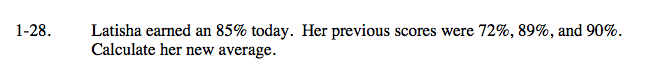### Home > CAAC > Chapter 1 > Lesson 1.1.3 > Problem1-28

1-28.Try not to be confused by the fact that the test scores are given in percentages. Treat them as you would any other values.

Calculate the average by dividng the sum of all the scores by the number of tests.

$\text{Average }= \frac{(72+89+90+85)}{4}=84\%$